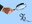# How is the interest in Kisan Vikas Patra calculated?

Understand the interest calculation and taxation under Kisan Vikas Patra (KVP) schemeKisan Vikas Patra (KVP)is a safe small-savings scheme provided by the Indian post office and backed by the Indian government. It is designed in such a way that the invested principal amount is doubled at the time of maturity. Hence, the duration is decided with respect to the interest rate. Right now, KVP is giving 6.90 per cent and accordingly the duration is 124 months (10 years four months). One of our readers wanted to know how the interest is calculated in KVP and how it is taxed.

The interest rate in the KVP is guaranteed at 6.90 per cent. These are compounded annually which means they are automatically reinvested and added to the principal every year. The rate is notified every quarter and the maturity period may change based on the rate. However, once you have invested, the rate will remain unchanged for you throughout the tenure.

There is no tax benefit on the deposit or the interest that the KVP earns. The interest earned on the KVP is added to your taxable income and taxed according to your tax slab. It is to note that KVP has a lock-in period of 30 months.

Let's understand how the interest is calculated with an example. Suppose you invested Rs 1 lakh in a KVP scheme with an annual return of 6.90 per cent. At the end of the first year, the interest earned would be Rs 6,900. This interest of Rs 6,900 is added to your income and taxed as per the slab you fall in. Since the interest is earned on a compounding basis, your principal at the beginning of the second year would be Rs 1,06,900. For the second year, you earn Rs 7,376. This is added to the first year's balance of Rs 1,06,900 and you end up with a total of Rs 1,14,276 at the end of the second year.

The same continues for the remaining duration of the scheme. Since the duration is 10 years and four months, after 10 years the interest is calculated only for four months which comes up to Rs 4,383. This essentially doubles your initial investment of Rs 1 lakh to Rs 2 lakh at maturity.

Everything about the National Savings Certificate scheme

An overview of Pradhan Mantri Vaya Vandana Yojana

## Recommended Stories

No change in interest rates of small-savings schemes